Journal of Integer Sequences, Vol. 2 (1999), Article 99.1.2

## Refactorable Numbers - A Machine Invention

### Simon Colton Mathematical Reasoning Group Institute of Representation and Reasoning Division of Informatics University of Edinburgh 80 South Bridge, Edinburgh, EH1 1HN SCOTLAND Email address: simonco@dai.ed.ac.uk

Abstract: The HR (or Hardy-Ramanujan) program invents and analyses definitions in areas of pure mathematics, including finite algebras, graph theory and number theory. While working in number theory, HR recently invented a new integer sequence, the refactorable numbers, which are defined and developed here. A discussion of how HR works, along with details of well known sequences reinvented by HR and other new sequences invented by HR is also given.

## 1. Introduction

The following sequence of integers has recently been accepted into the OnLine Encyclopedia of Integer Sequences, :

[A033950]: 1, 2, 8, 9, 12, 18, 24, 36, 40, 56, 60, 72, 80, 84, 88, 96, 104, 108, 128, 132, 136, 152, 156, ...

The sequence is interesting for two reasons:
• It has a simple definition: those integers n for which the number of divisors of n divides n.
• It was invented by a computer program.

This note gives some results about the refactorables, followed by a discussion of the HR program which invented them and a look at some of the other sequences HR has invented or reinvented.

## 2. Refactorable Numbers

Notation. Throughout, the number of divisors of an integer, n, is written τ(n), the sum of the divisors of n is written σ(n) and the number of integers less than and coprime to n is written φ(n).

Definition. An integer, n, is a refactorable number if and only if τ(n) divides n.

Lemma 1. (Theorem 273 from ) If the prime factorization of n is

n = p1m1 ... pkmk

then n has (m1 + 1) ... (mk + 1) divisors.

Lemma 2. An odd integer k is refactorable if and only if 2k is refactorable.

Proof. Suppose k = p1m1 ... pkmk   By Lemma 1, if k is refactorable, (m1 + 1) ... (mk + 1) divides k. Therefore the number of divisors of 2k, namely 2(m1 + 1)...(mk + 1), divides 2k, and so 2k is refactorable. The converse follows by reversing the steps of the argument. QED.

Theorem 1. There are infinitely many odd refactorable numbers, and infinitely many even refactorable numbers.

Proof. From Lemma 1, if p is a prime, q = pp - 1 has p divisors, and so q is refactorable. The result now follows from Lemma 2. QED.

The set of integers of the form pp - 1 forms a subsequence of the refactorable numbers:

[A036878]: 2, 9, 625, 117649, 25937424601, 23298085122481, 48661191875666868481, ...

A more interesting way to prove Theorem 1 is to take the integers in numerical order, find their prime factorizations and apply the map: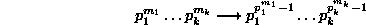For example:
3 = 31   becomes   331-1 = 9
and
6 = 2131   becomes   221-1331-1 = 18

It is easy to see that the integers produced from this map must all be refactorable numbers, as they have p1m1 ... pkmk divisors, and for any prime p and any integer m we have m <= pm-1, so the number of divisors divides the integer itself. The result of the transformation on a even number is another even number, and the result on an odd number is another odd number. It follows that there are infinitely many even and odd refactorable numbers.

This mapping of the integers onto the refactorables produces another interesting sequence:

[A036879]: 1, 2, 9, 8, 625, 18, 117649, 128, 6561, 1250, 25937424601, 56, 23298085122481, 235298, ...

This sequence does not of course include all refactorable numbers: it is easy to show that 12, for instance, does not belong to this sequence.

### 2.1 Membership Theorems

Since primes have two divisors, 2 is the only prime refactorable number.

Theorem 2. All odd refactorable numbers are squares.

Proof. Suppose n is as in Lemma 1, and is odd and refactorable. Since each mi + 1 must divide n, each mi is even, so n is a square. QED.

Theorem 2 makes it easy to search for odd refactorable numbers, which form this subsequence:

[A036896]: 1, 9, 225, 441, 625, 1089, 1521, 2025, 2601, 3249, 4761, 5625, 6561, 7569, 8649, ...

The odd numbers which square to give these are:

[A036897]: 1, 3, 15, 21, 25, 33, 39, 45, 51, 57, 69, 75, 81, 87, 93, ...

(It is easy to see that 3 is the only prime in this series.)

Theorem 3. No perfect number is refactorable.

Proof.
(a) Even perfect numbers. Using Theorem 277 from , we know that if k is an even perfect number, it has the form 2n-1(2n-1), where 2n-1 is a prime, p, and using Theorem 18 from , we know that if 2n-1 is prime, then so is n. Using Lemma 1, we know that k = 2n-1p must have ((n-1)+1)(1+1) = 2n divisors. If 2n divides k then either n = 2 or n = p (as n is a prime). If n = 2 then the perfect number is 6, which is not refactorable. If n = p then n = 2n-1, which is impossible for a prime n.
(b) Odd perfect numbers. No odd perfect numbers are known. If one were to exist, say q, with divisors d1 < ... < dk = q, then each di must be odd, and by definition, d1+ ... + dk-1 = q. The sum of an even number of odd integers is even, so, as q is odd, we know that k-1 must be odd, so q has an even number of divisors. Therefore q cannot be refactorable as it is odd and cannot be divisible by an even number. QED.

### 2.2 Pairs and Triples of Refactorable Numbers

Because odd refactorables are square numbers, we cannot have four or more consecutive refactorables, since positive squares always differ by more than 2. We cannot yet rule out triples of refactorable numbers, but we can show that it is very unlikely that they exist:

Theorem 4. If (a-1, a, a+1) is a triple of refactorable numbers, then a must be of the form: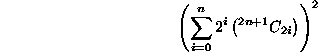for some integer n.

Proof. As odd refactorable numbers are square, and as no two square numbers differ by 2, a must be odd and a square, say b2. An instance of the Fermat-Euler theorem (Theorem 72 from ) states that b2 = 1 (mod 4), so b2 + 1 = 2 (mod 4). Therefore a + 1 is not divisible by 4 and so must have prime factorization a + 1 = 2 p1m1 ... pkmk, where the pi's are distinct odd primes. This means that τ(a+1) = 2(m1+1)...(mk+1) and that each mi+1 must be odd as a+1 is refactorable. So each mi must be even and therefore a+1 is twice an odd square number. Therefore we can write a+1 = 2c2, so b2 + 1 = 2c2. This means that (b,c) must be a solution of the Diophantine equation x2 - 2y2 = -1. Theorem 244 of  states that the positive integer solutions to this equation are given by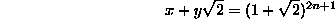for integers n. Expanding the coefficient of x on the right-hand side, we get: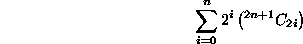and so a is as in the statement of the theorem. QED.

These numbers quickly become large. For example, if we take n = 10, then a = 2982076586042449. By considering n <= 35, it is easy to show that there are no triples between 1 and 1053, and it would not be difficult to take this number further.

Conjecture 1. There are no triples of refactorable numbers.

There are, however, pairs of refactorable numbers, although these are fairly rare. The only pairs of refactorable numbers between 1 and 1,000,000 are:

[A036898]: (1 2), (8 9), (1520 1521), (50624 50625), (62000 62001), (103040 103041),
(199808 199809), (221840 221841), (269360 269361), (463760 463761), (690560 690561),
(848240 848241), (986048 986049)

It is easy to see that if (a, a+1) is a pair of refactorable numbers, and a is even, then a is a multiple of four (from the Fermat-Euler theorem as in the proof of Theorem 4).

If two refactorables are relatively prime, their product is also refactorable. So the products of pairs of consecutive refactorables produces another (possibly finite) sequence of refactorables:

[A036899]: 2, 72, 2311920, 2562840000, 3844062000, 10617344640, 39923436672, ...

Conjecture 2. There are infinitely many pairs of refactorable numbers.

### 2.3 Distribution

We cannot yet give an accurate measure for the number of refactorables less than a given n, but we can say how many there are with a given number of divisors:

Theorem 5. The number of refactorable numbers with n divisors is:

• 1, if n = 1 or 4.
• k!, if n is the product of k distinct primes (ie. it is square-free:[A005117]).
• infinite, otherwise.

Proof. Clearly, 1 is the only refactorable number with one divisor. If an integer s has four divisors, then it must be of the form p3 or pq for distinct primes p, q. Taking the first case, if it is to be refactorable, then p = 2 and the refactorable number is 8. There are no refactorables of the form pq because 4 cannot divide the product of two distinct primes.
If n is the product of k distinct primes, n = p1 ... pk, then any integer s with n divisors must be of the form s = a1p1 - 1 ... akpk - 1 for distinct primes a1, ..., ak. If it is to be refactorable, then n must divide s, so {a1, ..., ak} = {p1, ..., pk} and there are k! ways to choose the ai's from the pi's, hence k! possibilities for s.
Suppose now that n is not square-free, and n = p1m1 ... pkmk. Firstly, if k = 1, then n = pm and m > 1 . Then for any prime q which is not p, the integer s = qp - 1ppm - 1 - 1 has n divisors. Further, s is refactorable unless

pm - 1 - 1 < m <=> p = m = 2 <=> n = 4 ,

and we have already dealt with the case where n = 4 . Secondly, if k > 1, with say mi > 1, for any prime q not in {p1, ..., pk}, the integerhas n divisors. Now s is also refactorable unless, as above, pi = mi = 2. If there is an i > 2 for which mi > 1, then choosing this i in the construction above works. This leaves only the case where n = 22 p2 ... pk. In this case, for any prime q not in {p1, ..., pk}, the integer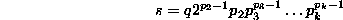has 2p2 2p3 ... pk = n divisors, and is refactorable because p2 > 2 implies p2 - 1 >= 2. QED.

Theorem 5 tells us, for instance, that there are precisely two refactorable numbers with 6 divisors, namely 12 and 18, and precisely 6 refactorable numbers with 30 divisors, namely 213254, 213452, 223154, 223451, 243152 and 243251.

Also, for a given non-square-free integer n = p1m1 ... pkmk , if we can write:

n = (m1+t1) ... (mk+tk) a1 ... aj

for j > 0, and some ti > 0, ai > 1, then for any set of primes, {q1, ..., qj}, none of which are in {p1, ..., pk}, the number: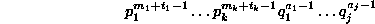will have n divisors and be refactorable.

So, for instance, because 36 = 22 32,

36 = (2+1)(2+1)4 implies 36p3 has 36 divisors and is refactorable (for any prime p > 3).
36 = (2+1)(2+1)2*2 implies 36pq has 36 divisors and is refactorable (for any primes p, q > 3).
36 = (2+1)(2+2)3 implies 108p2 has 36 divisors and is refactorable (for any prime p > 3).
36 = (2+2)(2+1)3 implies 72p2 has 36 divisors and is refactorable (for any prime p > 3).
36 = (2+1)(2+4)2 implies 972p has 36 divisors and is refactorable (for any prime p > 3).
36 = (2+4)(2+1)2 implies 288p has 36 divisors and is refactorable (for any prime p > 3).

Note that the first such formula comes from the first non-square-free integer greater than 4, namely 8, and we find that 8p is refactorable with 8 divisors, for any prime p > 2.

To end the discussion on refactorable numbers, we give a table of the distribution of (i) refactorable numbers, (ii) odd refactorable numbers, (iii) even refactorable numbers, (iv) pairs of refactorable numbers, and we compare these with the distribution of the primes and pairs of primes.

 n at most primes refactorables odd refactorables even refactorables prime pairs refactorable pairs 10 4 4 2 2 2 2 102 25 16 2 14 8 2 103 168 92 5 87 35 2 104 1229 665 15 650 205 3 105 9592 5257 34 5223 1224 5 106 78498 44705 87 44618 8169 13 107 664579 394240 237 394003 58980 27 108 5761455 ? 650 ? 440312 75 109 50847534 ? 1813 ? 3424506 187 1010 455052511 ? 5152 ? 27412679 468 1011 4118054813 ? 14889 ? 224376048 1219

Table 1: Distribution of refactorable numbers, odd and even refactorables and pairs of refactorables, compared with distribution of primes and prime pairs.

We used UBASIC and GAP to compile this table. Based on this empirical evidence, it appears that the number of refactorables is always at least half the number of primes. Using the prime number theorem, (Theorem 6 of ), we can conjecture that the number of refactorables less than x is at least x/(2 log(x)).

## 3. HR - Automatic Concept Formation

The research of the author includes understanding and automating the processes at work when mathematicians invent new concepts, specifically in finite group theory. This has culminated in the HR system, named after Hardy and Ramanujan, to emphasize both a theory-driven and a data-driven approach to concept formation. HR starts with only the axioms of group theory and ends with definitions and models of concepts it has derived, such Abelian groups, cyclic groups, orders of elements and so on. It does this by:

1. Finding models of groups using the MACE model finder, .
2. Storing data from the group tables which details the core concepts in group theory, namely the group operation, the identity element and the inverses of elements.
3. Manipulating this data in one of eight ways to produce a new data-table from one (or two) old ones.
4. Assigning definitions to each new data-table using the information about how they were constructed.

Most of the concepts HR invents are calculations which can be made directly from the group table of a finite group. However, it also makes sequences of groups, for instance, the sequence formed by taking the subgroup generated by the center of the previous group (which produces sequences of length two only). The sequences produced were mostly disappointing. For this reason, we looked towards number theory to see if it was possible to find more interesting sequences using HR's limited set of production rules.

### 3.1 HR Working in Number Theory

In number theory, HR generated three initial tables for the integers up to 100:

• The set of triples [a, b, c] for which a = b * c.
• The set of triples [a, b, c] for which a = b + c.
• The set of pairs [a, b] for which b is a digit in the decimal expansion of a.
These were quite arbitrary choices - other choices would lead to different concepts. HR performs concept formation in number theory using the same manipulations as in group theory. It also has three ways to produce sequences of numbers:
1. By taking the sequence of integers with a given property, e.g. the prime numbers.
2. By taking the output of some function on successive integers, e.g. the τ function.
3. By finding those integers which, for some function, output an integer larger than any output for a smaller integer, i.e. those integers which set a record, such as the highly composite numbers.

The first time HR was tried in number theory, it invented the refactorable numbers. When we first saw this sequence, we did not know how it was found, but it looked interesting - it had a mix of odd and even numbers, sufficiently many terms between one and a hundred, and no obvious pattern. Therefore we looked it up in the Online Encyclopedia, and were surprised to find that it was not listed. Only then did we look at the output from HR to see its definition (expecting an unintuitive, complicated explanation), and were then even more surprised that this sequence was missing from the Encyclopedia.

We must point out that HR did only the easy part - it invented the concept - we have done all the rest of the above work. However, HR does make conjectures about refactorables. For example, it made the following conjecture, which we thought was true until a very large counterexample was found:

Conjecture. Given a refactorable number n, let

f(n) = |{(a,b) in N × N : ab = n, a =\= b}| .

Then f(n) is not a divisor of n if and only if n is a square number.

Proof of [==>] Suppose n is refactorable, that f(n) does not divide n and that n is not a square. Then f(n) is equal to the number of divisors of n, and so must divide n, a contradiction.
Disproof of [<==] 36360900, 79388100 and 155600676 are the first three square refactorable number which are divisible by f(n).

Since HR only knew the factorizations of the integers up to 100, the conjecture was not implausible.

Note that there can be no odd refactorable numbers n for which f(n) divides n, because if n is odd and refactorable, it must be a square, and if so, f(n) is one less than the number of divisors of n, so, as n is refactorable, f(n) + 1 must divide n, and as n is odd, we cannot have both f(n) and f(n) + 1 dividing n, as one of these must be even.

We note that HR has in effect discovered the concept of square numbers, for which both τ(n) and τ(n)-1 divide n.

The odd or even square refactorable numbers are:

[A036907]: 1, 9, 36, 225, 441, 625, 1089, 1521, 2025, 2601, 3249, 3600, 4761, 5625, 6561, 7569, 8100, ...

### 3.2.1 Data Mining Using the Encyclopedia

Having down-loaded a copy of the Online Encyclopedia, we have enabled HR to check each sequence it makes against the database and flag those which it has reinvented. After tidying up the data, we were also able to write an add-on program enabling HR to perform some data mining with the encyclopedia. We are still implementing, experimenting and collating results, but it seems that it is certainly possible to find previously unknown results using data mining. For example, we asked HR to identify any sequences for which the refactorables are a subsequence. It first found [A009230], in which the nth term is lcm(n, d(n)), which was not too surprising since for every refactorable number r, lcm(r, τ(r)) = r.

Next, HR spotted that the refactorables are a subsequence of [A047466], the integers congruent to 0, 1, 2 or 4 (mod 8). This was an unknown result, which we subsequently proved:

Theorem 6. Refactorable numbers are congruent to 0, 1, 2 or 4 (mod 8).

Proof. Odd refactorables are squares, and therefore congruent to 1 modulo 8. If a refactorable number were congruent to 6 mod 8 then it would be of the form 2(4n+3), and by Lemma 2, 4n+3 would also be refactorable, a contradiction. QED.

This gives us another insight into triples of refactorables:

Corollary. If (a-1, a, a+1) is a triple of refactorable numbers, then a = b2 for some odd number b and b2 = 16c + 1 for some c.

Proof. By Theorem 6, odd refactorables are congruent to 1 (mod 8), hence a+1 = 8n + 2 = 2(4n + 1) for some n. Therefore, as a + 1 is refactorable and 4n + 1 is odd, we see by Lemma 2 that 4n + 1 is an odd refactorable number. Hence, by Theorem 6 again, 4n + 1 = 8c+1, so a + 1 = 2(8c+1) and we see that a = 16c+1. As a is an odd refactorable, by Theorem 2 we can write a = b2 for some b, and a = b2 = 16c+1. QED.

Another data mining investigation using the encyclopedia, this time to find super-sequences of the perfect numbers ["even" being understood here], showed that perfect numbers are a subsequence of [A009242], with nth term equal to lcm(n, σ(n)). Therefore HR had spotted that, for all (even) perfect numbers p, there is an n such that lcm(n, σ(n)) = p. In the same session, HR also spotted that the even perfect numbers are a subsequence of [A007517], in which the nth term is φ(n)(σ(n)-n). We have subsequently proved both of these results:

Theorem 7. For any even perfect number p, there is an integer a for which lcm(a, σ(a)) = p, and an integer b for which φ(b)(σ(b)-b) = p.

Proof. From Theorem 277 of , we note that p = 2n - 1 (2n-1) for some n, where 2n-1 is a prime. If we take a = 2n-1 then

σ(a) = 1 + 2 + ... + 2n-1 = 2n - 1
and so lcm(a, σ(a)) = 2n-1 (2n-1) = p, because 2n-1 is prime.
If we take b = 2n then σ(b) = 2n + 1-1 and only the odd integers less than b will be coprime to it, so
φ(b) = b/2 = 2n-1
Therefore
φ(b)(σ(b) - b) = 2n-1 (2n + 1 - 1 - 2n) = 2n - 1 (2n - 1) = p.
QED.

So HR has highlighted the following appealing parallel between the refactorable numbers and the even perfect numbers:

• Refactorable numbers are of the form lcm(a, τ(a)) for some a.
• Perfect numbers are of the form lcm(a, σ(a)) for some a.
We also note that
• Refactorable numbers are those n for which lcm(n, τ(n)) = n,
• Perfect numbers are those n for which lcm(n, σ(n)) = 2n.

### 3.2.2 A Mathematical Cycle

We have recently been able to complete a cycle of mathematical activities by using the automated theorem prover OTTER  to prove some of the conjectures that HR makes in group theory. HR now:
• Invents definitions
• Spots conjectures involving those definitions
• Proves some of the conjectures using OTTER
• Finds counterexamples to some of the conjectures that OTTER fails to prove
Therefore, starting with only the axioms of group theory, HR constructs a theory with (i) examples of groups, (ii) definitions of groups and models of those definitions, (iii) proven conjectures with proofs supplied by OTTER, (iv) open conjectures, where OTTER has failed to find a proof and MACE has failed to find a counterexample.

 gives a more detailed description of the HR system, and the following web page is devoted to HR:

http://dream.dai.ed.ac.uk/group/simonco/hr/

## 4. Other Sequences

### 4.1 Re-Invented Sequences

After a preliminary examination, we can claim that HR reinvented the following sequences:

1. Sequences resulting from factorization:
• the square numbers [A000290].
• the non-squares [A000037].
• the prime numbers [A000040].
• the squares of primes [A001248].
• the primes and squares of primes [A000430].
• 1 and the odd primes [A006005].
• 0 if prime, 1 otherwise [A005171].
• the composite numbers [A002808].
• the highly composite numbers [A002182].
• τ(n), the number of divisors of n [A000005].
• the number of proper divisors [A032741].
• integers with 4 [A030513], 5 [A030514], etc. divisors.
• integer square roots or zero [A037213].
• writing out the divisors [A027750].
• writing out the proper divisors [A027751].
• the squarefree integers. [A005117].
• the powers of 2 [A000079].
• integers with at most 2 prime factors [A037143].
• integers not divisible by 3 [A001651].

• the even numbers [A005843].
• the odd numbers [A005408].
• writing out the numbers less than n [A005408].
• 1 together with the even numbers [A004277].
• 2 together with the odd numbers [A004280].
• 2,4 and the odd numbers [A004281].

3. Sequences resulting from examination of digits:
• the repunits [A000042].
• integers with only 2's as digits [A002276].
• integers with a 1 [A011531], 2 [A011532], 3 [A011533], etc. as a digit.
• the repdigits [A010785].
• integers divisible by each non-zero digit [A002796].
• each digit is prime [A046034].
• the numbers with two distinct digits [A031955].
• the number of distinct digits in n [A043537].
• number with distinct digits [A010784].
• numbers divisible by every digit [A034838].
• no base 2 digit is a base 10 digit [A037344].
• the natural numbers in base 3 [A007089].

More interesting than the fact that HR reinvented these sequences are the ways in which HR defines them. For example, even numbers are defined as integers n for which there is a natural number m such that m + m = n. The natural numbers in base 3 were defined as integers in base 10 which have no digits which can be written as a + b where a > 0, b > 0 and a =/= b. Powers of two were defined as those integers with no odd divisors.

### 4.2 New Sequences Invented by HR

HR invented many other sequences which were not found in the encyclopedia. Most did not seem of any great interest. However, we deemed the following seven of sufficient interest to be submitted to the encyclopedia.

1. 1, 2, 14, 23, 29, 34, 46, 63, 68, 74, 76, 78, 88, 94, ... [A036433],
the number of divisors is a digit in the decimal expansion of n. This sequence contains all primes with a 2 in them, and those primes starting with a 2, [A045708].

2. 1, 4, 9, 11, 14, 19, 41, 44, 49, 91, 94, 99, ... [A036435],
those integers where all the digits are nonzero squares.

3. 0, 1, 0, 1, 1, 0, 2, 0, 1, 1, 0, 2, 1, 1, 1, 0, 0, ... [A036431],
f(n) = |{a < n : a + τ(a) = n}|.

4. 1, 3, 6, 8, 11, 16, 17, 20, 22, 23, 27, 29, ... [A036434],
integers which cannot be written as a + τ(a) for some a (i.e. those n for which f(n) = 0).

5. 1, 2, 7, 38, 122, 2766, 64686, ... [A036432],
integers that set a record for f(n).

6. 1, 4, 6, 10, 12, 14, 22, 24, 26, 27, ... [A036438],
integers which can be written as m * τ(m) for some m (which of course include twice the primes, [A001747]).

7. 1, 6, 8, 10, 14, 15, 22, 26, 27, ... [A036436],
integers for which τ(n) is a square. This sequence contains the multiplicatively perfect numbers [A007422], n such that the product of the divisors of n equals n2.

HR has also found some interesting finite sequences of integers. For example, HR invented those integers which have more distinct digits than any smaller number

1, 10, 102, 1023, 10234, 102345, 1023456, 10234567, 102345678, 1023456789 [A038378].

## 5. Conclusions

We have shown that refactorable numbers are of some interest, and hope that someone will take up the challenge of proving the conjectures from this paper (before we do).

Also, we have shown that HR is capable of producing interesting concepts. Automated concept formation programs have some advantages over humans, in that they have no pride (are not ashamed to look at concepts with simple descriptions) and are very thorough. The use of computers with integer sequences has also been explored in , where the Seek-Whence program was used to identify definitions of integer sequences. Indeed, the Superseeker server for the Online Encyclopedia of Integer Sequences does a certain amount of concept formation in attempting to find a match between a given sequence and one in the database. A similar program to HR is Graffiti, , which makes conjectures in graph theory. Another program, , automatically invents theorems in plane geometry.

Machine discovery in mathematics and in science in general is a productive and interesting area which is gaining recognition and attention. HR and refactorable numbers were themselves recently mentioned in the popular press, , which reflects the interest that machine discovery is attracting. As more work is done in this area, we hope to make discovery programs as much a part of the mathematician's tool box as computer algebra packages.

## 6. Acknowledgements

This work is supported by EPSRC research grant GR/L 11724. I would like to thank Prof. Alan Bundy and Dr. Toby Walsh (who gave refactorables their name) for their valuable contributions to this work.

## 7. References

 Bagai, R., Shanbhogue, V., Zytkow, J. M. and Chou, S. C.: Automatic theorem generation in plane geometry, in Lecture Notes in Artificial Intelligence, Vol. 689, Springer-Verlag, 1993.

 Fajtlowicz, S.: On conjectures of Graffiti: Discrete Mathematics, Vol. 72, pages 113-118, 1988. (See also Fajtlowicz, S.: On conjectures of Graffiti V. In Proceedings of the 7th International Quadrennial Conference on Graph Theory, Combinatorics and Applications, Vol. 1, pages 367-376, 1995.)

 Sloane, N. J. A.: The On-Line Encyclopedia of Integer Sequences, published electronically at http://oeis.org.

## 8. Addenda, April 19, 1999

In this paper we tried to make two points: (i) that the HR program we have implemented can invent new and interesting concepts in number theory and (ii) that one concept output by HR, namely the refactorable numbers, had many interesting properties. Because refactorables and associated sequences were missing from the Encyclopedia of Integer Sequences, , and after a preliminary search of the relevant literature, we concluded that the refactorable numbers were a genuinely new invention.

However, on 23rd March 1999, we received notification from Robert Kennedy and Curtis Cooper, of Central Missouri State University, that they had read the above paper and that the concept of refactorables had been defined already as `τ numbers' in a 1990 paper, , which proved that the natural density of the τ numbers is zero.

This news detracts from our original paper in only one way, namely that the title is inaccurate, refactorables were a machine re-invention. Indeed, the news that they have been developed so recently adds both to the point that HR can invent and re-invent concepts of interest and, of course, to the point that refactorables are interesting.

To add to the argument that HR produces interesting, novel concepts in number theory, we present the following very simple function defined by HR recently:

f(n) = |{(a,b) : a * b = n and a | b}|.

This has been added to the encyclopedia:

[A046951]: 1, 1, 1, 2, 1, 1, 1, 2, 2, 1, 1, 2, 1, 1, 1, 3, 1, 2, 1, 2, 1, 1, 1, 2, 2, 1, 2, 2, 1, 1, 1, 3, 1, 1, 1, 4, 1, ...

This was interesting because of its similarity to the τ function, [A000005]: (τ(n) = number of divisors of n). HR went on to define the integer sequence of numbers which set a record for f (ie. those integers, a, for which for all b, 0 < b < a, f(a) > f(b)):

[A046952]: 1, 4, 16, 36, 144, 576, 1296, 2304, 3600, 14400, 32400, 57600, 129600, 518400, ...

It was easy to spot that these are all square numbers, but a little investigating revealed the following result:

Theorem 1. The nth integer setting the record for f as above is the square of the nth highly composite number [A002182] (the highly composite numbers have more divisors than any smaller integer).

To prove this, we need the following lemma:

Lemma 1. f(n) = τ(square root of the largest square dividing n). Note that the square root of the largest square dividing n, which we write as s(n), is integer sequence [A000188].

Proof of Lemma 1.
Writing n=p1k1...pmkm, the largest square dividing n is p12[k1/2]...pm2[km/2], the square root of which is: p1[k1/2]...pm[km/2], where [z] denotes the integer part of fraction z. The pairs of integers dividing n are of the form:

(a,b) = (p1x1...pmxm, p1k1 - x1...pmkm - xm)

and a|b if for all i, xi =< ki - xi, ie. xi =< ki/2. Therefore, each xi can be 0,1,2,...,[ki/2], and:

f(n) = ([k1/2] + 1)([k2/2] + 1)...([km/2] + 1) = τ(p1[k1/2]...pm[k1/2]) = τ(s(n)),

using Theorem 273 from . QED

Proof of Theorem 1.
Suppose that a sets a record for f. Therefore, f(a) > f(1), f(a) > f(2), ..., f(a) > f(a-1), and by lemma 1, this means that τ(s(a)) > τ(s(1)), τ(s(a)) > τ(s(2)), ..., τ(s(a)) > τ(s(a-1)). Suppose now that c2 is the largest square less than or equal to a. Then we see that:

τ(s(a)) > τ(s(1)) = τ(1)
τ(s(a)) > τ(s(4)) = τ(2)
.     .
τ(s(a)) > τ(s((c-1)2)) = τ(c-1)

If a > c2, the largest square dividing a will be less than c2 and s(a) < c. But then, s(a) = c - k for some k, and τ(s(a)) = τ(c-k), which is a contradiction. Hence a = c2, s(a) = c, and τ(c) > τ(c - i) for all i < c, which makes c a highly composite number, and a the square of a highly composite number.

Suppose now that b is a highly composite number, and a=b2. Therefore, s(a) = b, and, as b is highly composite, if, for some k, τ(s(a-k)) > τ(s(a)), then s(a-k) > s(a) = b, which is impossible. Hence, for all k < a, τ(s(a)) > τ(s(a - k)), and by lemma 1, f(a) > f(a - k), thus a sets a record for f. QED

Coincidentally, the previous time there was a similar confusion over a machine invented concept, Douglas Lenat, who wrote the AM program, , thought for a while that what he called maximally divisible numbers were a machine invention. These turned out to be the highly composite numbers we've discussed here, which were originally developed by Ramanujan, .

This time, we will only claim that it is possible that the function f above was invented by HR, and that it is possible that, while the concept of the squares of highly composite numbers may have been looked at before, HR may have been the first to define them as setting the record for f. It was fortunate that τ numbers had not been added to the encyclopedia, because we may have decided not to develop them. However, the story of refactorable/τ numbers emphasises the need for databases of mathematical knowledge such as the Encyclopedia of Integer Sequences, , which can be used by people and computers alike to check the novelty of inventions.